Anúncio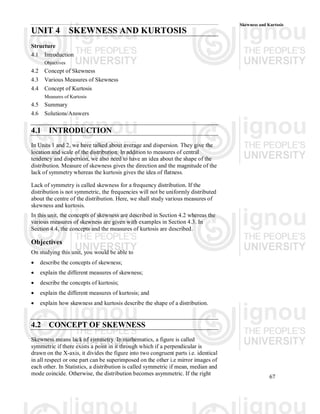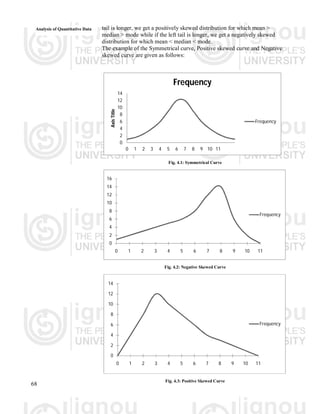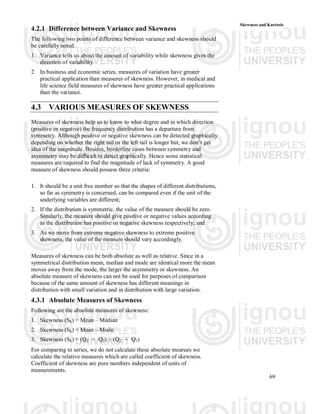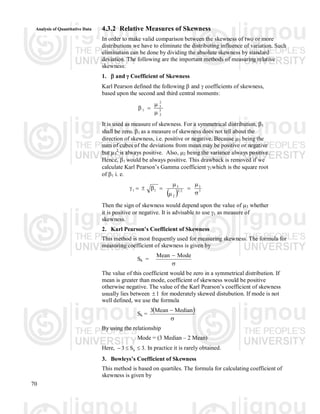Anúncio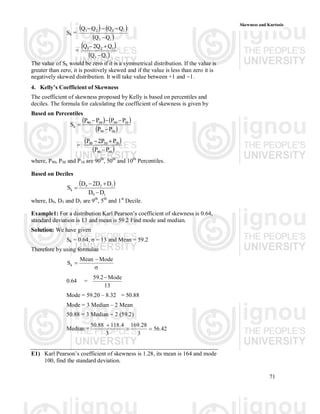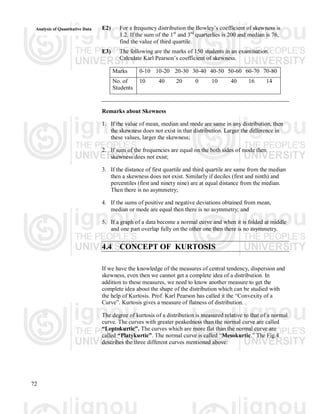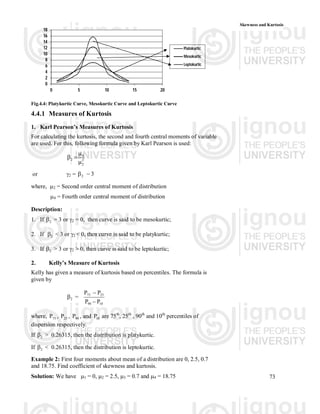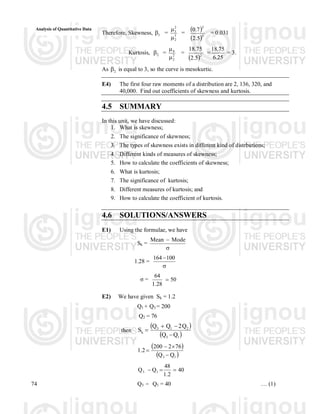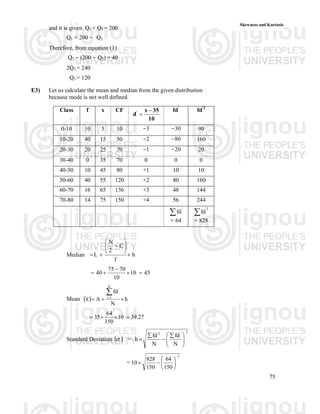Anúncio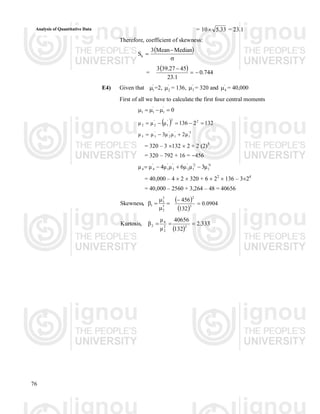Próximos SlideSharesSkewness
Carregando em ... 3
1 de 10
Anúncio

### Skewness.pdf

1. 67 Skewness and Kurtosis UNIT 4 SKEWNESS AND KURTOSIS Structure 4.1 Introduction Objectives 4.2 Concept of Skewness 4.3 Various Measures of Skewness 4.4 Concept of Kurtosis Measures of Kurtosis 4.5 Summary 4.6 Solutions/Answers 4.1 INTRODUCTION In Units 1 and 2, we have talked about average and dispersion. They give the location and scale of the distribution. In addition to measures of central tendency and dispersion, we also need to have an idea about the shape of the distribution. Measure of skewness gives the direction and the magnitude of the lack of symmetry whereas the kurtosis gives the idea of flatness. Lack of symmetry is called skewness for a frequency distribution. If the distribution is not symmetric, the frequencies will not be uniformly distributed about the centre of the distribution. Here, we shall study various measures of skewness and kurtosis. In this unit, the concepts of skewness are described in Section 4.2 whereas the various measures of skewness are given with examples in Section 4.3. In Section 4.4, the concepts and the measures of kurtosis are described. Objectives On studying this unit, you would be able to  describe the concepts of skewness;  explain the different measures of skewness;  describe the concepts of kurtosis;  explain the different measures of kurtosis; and  explain how skewness and kurtosis describe the shape of a distribution. 4.2 CONCEPT OF SKEWNESS Skewness means lack of symmetry. In mathematics, a figure is called symmetric if there exists a point in it through which if a perpendicular is drawn on the X-axis, it divides the figure into two congruent parts i.e. identical in all respect or one part can be superimposed on the other i.e mirror images of each other. In Statistics, a distribution is called symmetric if mean, median and mode coincide. Otherwise, the distribution becomes asymmetric. If the right
2. Analysis of Quantitative Data 68 tail is longer, we get a positively skewed distribution for which mean > median > mode while if the left tail is longer, we get a negatively skewed distribution for which mean < median < mode. The example of the Symmetrical curve, Positive skewed curve and Negative skewed curve are given as follows: 0 2 4 6 8 10 12 14 0 1 2 3 4 5 6 7 8 9 10 11 Axis Title Frequency Frequency Fig. 4.1: Symmetrical Curve 0 2 4 6 8 10 12 14 16 0 1 2 3 4 5 6 7 8 9 10 11 Frequency Fig. 4.2: Negative Skewed Curve 0 2 4 6 8 10 12 14 0 1 2 3 4 5 6 7 8 9 10 11 Frequency Fig. 4.3: Positive Skewed Curve
3. 69 Skewness and Kurtosis 4.2.1 Difference between Variance and Skewness The following two points of difference between variance and skewness should be carefully noted. 1. Variance tells us about the amount of variability while skewness gives the direction of variability. 2. In business and economic series, measures of variation have greater practical application than measures of skewness. However, in medical and life science field measures of skewness have greater practical applications than the variance. 4.3 VARIOUS MEASURES OF SKEWNESS Measures of skewness help us to know to what degree and in which direction (positive or negative) the frequency distribution has a departure from symmetry. Although positive or negative skewness can be detected graphically depending on whether the right tail or the left tail is longer but, we don’t get idea of the magnitude. Besides, borderline cases between symmetry and asymmetry may be difficult to detect graphically. Hence some statistical measures are required to find the magnitude of lack of symmetry. A good measure of skewness should possess three criteria: 1. It should be a unit free number so that the shapes of different distributions, so far as symmetry is concerned, can be compared even if the unit of the underlying variables are different; 2. If the distribution is symmetric, the value of the measure should be zero. Similarly, the measure should give positive or negative values according as the distribution has positive or negative skewness respectively; and 3. As we move from extreme negative skewness to extreme positive skewness, the value of the measure should vary accordingly. Measures of skewness can be both absolute as well as relative. Since in a symmetrical distribution mean, median and mode are identical more the mean moves away from the mode, the larger the asymmetry or skewness. An absolute measure of skewness can not be used for purposes of comparison because of the same amount of skewness has different meanings in distribution with small variation and in distribution with large variation. 4.3.1 Absolute Measures of Skewness Following are the absolute measures of skewness: 1. Skewness (Sk) = Mean – Median 2. Skewness (Sk) = Mean – Mode 3. Skewness (Sk) = (Q3 - Q2) - (Q2 - Q1) For comparing to series, we do not calculate these absolute mearues we calculate the relative measures which are called coefficient of skewness. Coefficient of skewness are pure numbers independent of units of measurements.
4. Analysis of Quantitative Data 70 4.3.2 Relative Measures of Skewness In order to make valid comparison between the skewness of two or more distributions we have to eliminate the distributing influence of variation. Such elimination can be done by dividing the absolute skewness by standard deviation. The following are the important methods of measuring relative skewness: 1.  and  Coefficient of Skewness Karl Pearson defined the following  and  coefficients of skewness, based upon the second and third central moments: 3 2 2 3 1     It is used as measure of skewness. For a symmetrical distribution, 1 shall be zero. 1 as a measure of skewness does not tell about the direction of skewness, i.e. positive or negative. Because 3 being the sum of cubes of the deviations from mean may be positive or negative but 3 2 is always positive. Also, 2 being the variance always positive. Hence, 1 would be always positive. This drawback is removed if we calculate Karl Pearson’s Gamma coefficient 1which is the square root of 1 i. e.   3 3 2 3 2 3 1 1           Then the sign of skewness would depend upon the value of 3 whether it is positive or negative. It is advisable to use 1 as measure of skewness. 2. Karl Pearson’s Coefficient of Skewness This method is most frequently used for measuring skewness. The formula for measuring coefficient of skewness is given by Sk =   Mode Mean The value of this coefficient would be zero in a symmetrical distribution. If mean is greater than mode, coefficient of skewness would be positive otherwise negative. The value of the Karl Pearson’s coefficient of skewness usually lies between 1  for moderately skewed distubution. If mode is not well defined, we use the formula Sk =     Median Mean 3 By using the relationship Mode = (3 Median – 2 Mean) Here, . 3 S 3 k    In practice it is rarely obtained. 3. Bowleys’s Coefficient of Skewness This method is based on quartiles. The formula for calculating coefficient of skewness is given by
5. 71 Skewness and Kurtosis Sk =       1 3 1 2 2 3 Q Q Q Q Q Q     =     1 3 1 2 3 Q Q Q Q 2 Q    The value of Sk would be zero if it is a symmetrical distribution. If the value is greater than zero, it is positively skewed and if the value is less than zero it is negatively skewed distribution. It will take value between +1 and -1. 4. Kelly’s Coefficient of Skewness The coefficient of skewness proposed by Kelly is based on percentiles and deciles. The formula for calculating the coefficient of skewness is given by Based on Percentiles       10 90 10 50 50 90 k P P P P P P S      =     10 90 10 50 90 P P P P 2 P    where, P90, P50 and P10 are 90th , 50th and 10th Percentiles. Based on Deciles   1 9 1 5 9 k D D D D 2 D S     where, D9, D5 and D1 are 9th , 5th and 1st Decile. Example1: For a distribution Karl Pearson’s coefficient of skewness is 0.64, standard deviation is 13 and mean is 59.2 Find mode and median. Solution: We have given Sk = 0.64, σ = 13 and Mean = 59.2 Therefore by using formulae    Mode Mean Sk 0.64 = 13 Mode 2 . 59  Mode = 59.20 – 8.32 = 50.88 Mode = 3 Median – 2 Mean 50.88 = 3 Median - 2 (59.2) Median = 42 . 56 3 28 . 169 3 4 . 118 88 . 50    E1) Karl Pearson’s coefficient of skewness is 1.28, its mean is 164 and mode 100, find the standard deviation.
6. Analysis of Quantitative Data 72 E2) For a frequency distribution the Bowley’s coefficient of skewness is 1.2. If the sum of the 1st and 3rd quarterlies is 200 and median is 76, find the value of third quartile. E3) The following are the marks of 150 students in an examination. Calculate Karl Pearson’s coefficient of skewness. Marks 0-10 10-20 20-30 30-40 40-50 50-60 60-70 70-80 No. of Students 10 40 20 0 10 40 16 14 Remarks about Skewness 1. If the value of mean, median and mode are same in any distribution, then the skewness does not exist in that distribution. Larger the difference in these values, larger the skewness; 2. If sum of the frequencies are equal on the both sides of mode then skewness does not exist; 3. If the distance of first quartile and third quartile are same from the median then a skewness does not exist. Similarly if deciles (first and ninth) and percentiles (first and ninety nine) are at equal distance from the median. Then there is no asymmetry; 4. If the sums of positive and negative deviations obtained from mean, median or mode are equal then there is no asymmetry; and 5. If a graph of a data become a normal curve and when it is folded at middle and one part overlap fully on the other one then there is no asymmetry. 4.4 CONCEPT OF KURTOSIS If we have the knowledge of the measures of central tendency, dispersion and skewness, even then we cannot get a complete idea of a distribution. In addition to these measures, we need to know another measure to get the complete idea about the shape of the distribution which can be studied with the help of Kurtosis. Prof. Karl Pearson has called it the “Convexity of a Curve”. Kurtosis gives a measure of flatness of distribution. The degree of kurtosis of a distribution is measured relative to that of a normal curve. The curves with greater peakedness than the normal curve are called “Leptokurtic”. The curves which are more flat than the normal curve are called “Platykurtic”. The normal curve is called “Mesokurtic.” The Fig.4 describes the three different curves mentioned above:
7. 73 Skewness and Kurtosis 0 2 4 6 8 10 12 14 16 18 0 5 10 15 20 Platokurtic Mesokurtic Leptokurtic Fig.4.4: Platykurtic Curve, Mesokurtic Curve and Leptokurtic Curve 4.4.1 Measures of Kurtosis 1. Karl Pearson’s Measures of Kurtosis For calculating the kurtosis, the second and fourth central moments of variable are used. For this, following formula given by Karl Pearson is used: 2 2 4 2     or 2 = 3 2   where, 2 = Second order central moment of distribution 4 = Fourth order central moment of distribution Description: 1. If 2  = 3 or 2 = 0, then curve is said to be mesokurtic; 2. If 2  < 3 or 2 < 0, then curve is said to be platykurtic; 3. If 2  > 3 or 2 > 0, then curve is said to be leptokurtic; 2. Kelly’s Measure of Kurtosis Kelly has given a measure of kurtosis based on percentiles. The formula is given by 2  = 10 90 25 75 P P     where, 75  , 25  , 90  , and 10  are 75th , 25th , 90th and 10th percentiles of dispersion respectively. If 2  > 0.26315, then the distribution is platykurtic. If 2  < 0.26315, then the distribution is leptokurtic. Example 2: First four moments about mean of a distribution are 0, 2.5, 0.7 and 18.75. Find coefficient of skewness and kurtosis. Solution: We have 1 = 0, 2 = 2.5, 3 = 0.7 and 4 = 18.75
8. Analysis of Quantitative Data 74 Therefore, Skewness, 1  = 3 2 2 3   =    3 2 5 . 2 7 . 0 = 0.031 Kurtosis, 2  = 2 2 4   =  2 5 . 2 75 . 18 = 25 . 6 75 . 18 = 3. As 2  is equal to 3, so the curve is mesokurtic. E4) The first four raw moments of a distribution are 2, 136, 320, and 40,000. Find out coefficients of skewness and kurtosis. 4.5 SUMMARY In this unit, we have discussed: 1. What is skewness; 2. The significance of skewness; 3. The types of skewness exists in different kind of distrbutions; 4. Different kinds of measures of skewness; 5. How to calculate the coefficients of skewness; 6. What is kurtosis; 7. The significance of kurtosis; 8. Different measures of kurtosis; and 9. How to calculate the coefficient of kurtosis. 4.6 SOLUTIONS/ANSWERS E1) Using the formulae, we have Sk =   Mode Mean 1.28 =  100 164 σ = 50 28 . 1 64  E2) We have given Sk = 1.2 Q1 + Q3 = 200 Q2 = 76 then     1 3 2 1 3 k Q Q Q 2 Q Q S         1 3 Q Q 76 2 200 1.2     40 2 . 1 48 Q Q 1 3    Q3 - Q1 = 40 … (1)
9. 75 Skewness and Kurtosis and it is given Q1 + Q3 = 200 Q1 = 200 - Q3 Therefore, from equation (1) Q3 - (200 - Q3) = 40 2Q3 = 240 Q3 = 120 E3) Let us calculate the mean and median from the given distribution because mode is not well defined. Class f x CF 10 35 x d'   fd' fd'2 0-10 10 5 10 -3 -30 90 10-20 40 15 50 -2 -80 160 20-30 20 25 70 -1 -20 20 30-40 0 35 70 0 0 0 40-50 10 45 80 +1 10 10 50-60 40 55 120 +2 80 160 60-70 16 65 136 +3 48 144 70-80 14 75 150 +4 56 244 ' fd  = 64 2 ' fd  = 828 Median h f C 2 N L             45 10 10 70 75 40      Mean   h N fd A x k 1 i '      27 . 39 10 150 64 35     Standard Deviation    = 2 ' 2 ' N fd N fd h             = 2 150 64 150 828 10        
10. Analysis of Quantitative Data 76 = 33 . 5 10 = 23.1 Therefore, coefficient of skewness:      Median Mean 3 Sk =   744 . 0 1 . 23 45 27 . 39 3    E4) Given that ' 1  =2, ' 2  = 136, ' 3  = 320 and ' 4  = 40,000 First of all we have to calculate the first four central moments 0 ' 1 ' 1 1         132 2 136 µ µ µ 2 2 ' 1 ' 2 2      3 ' 1 ' 1 ' 2 ' 3 3 µ 2 µ µ 3 µ µ    = 320 – 3 132  2 + 2 (2)3 = 320 – 792 + 16 = -456 4 ' 1 2 ' 1 ' 2 ' 3 ' 1 ' 4 4 µ 3 µ µ 6 µ µ 4 µ µ     = 40,000 – 4  2  320 + 6  22  136 – 324 = 40,000 – 2560 + 3,264 – 48 = 40656      3 2 2 3 1 , Skewness       3 2 132 456 0904 . 0   333 . 2 132 40656 µ µ β , Kurtosis 2 2 2 4 2   
Anúncio